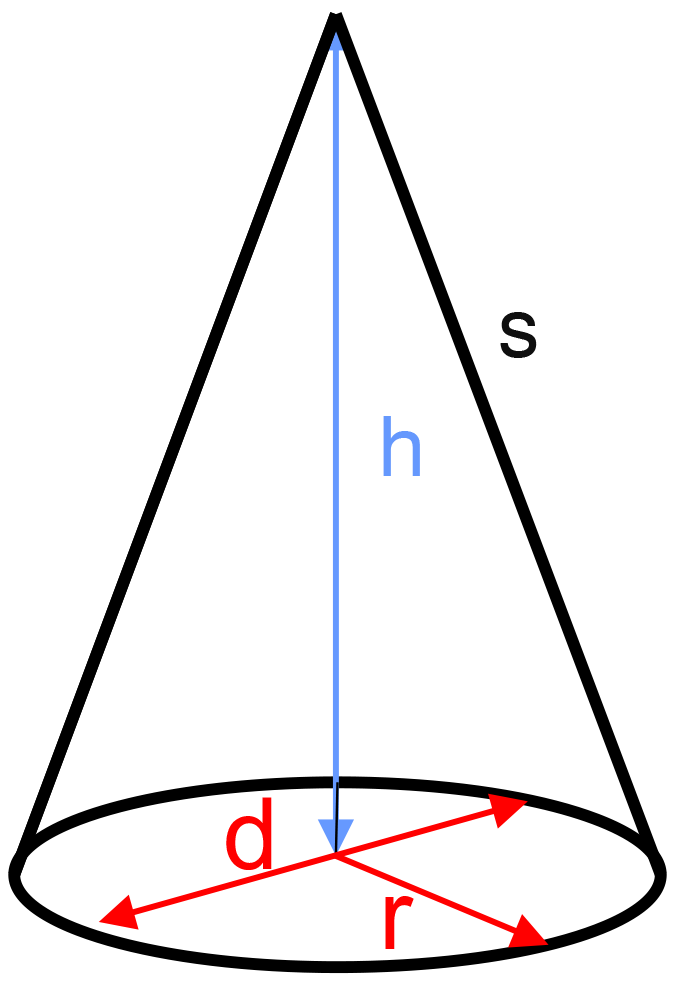﻿ Cone: - on line calculation, formula - FORMIAX

# Cone - surface and volumeS = πr ( r + s )

V = 1/3 πr2v

S = surface area

V = volume

d = diameter

h = height

s = slant height

WHAT IS IT ?
Cone is solid (3-dimensional) object with a circular flat base joined to a curved side that ends in an apex point.

CALCULATION:

Enter unit e.g.: inch

Enter height "h"

Round to number of decimals places

YOU MIGHT BE INTERESTED: